# 1 Handling skew distributions and/or outliers

Figure 1.1 plots heart weight against body weight, for 97 male cats. A regression line has been fitted.

cap1 <- "Heart weight versus body weight of 97 male cats.
A regression line has been fitted."
cap2 <- "Q-Q plot ('Normal Probability plot') of residuals from
the regression of heart weight on body weight, for the male cats."
cap3 <- "Scatterplot matrix of bootstrap estimates of Slope
versus Intercept, with boxplots used to summarize the marginal
distributions."
cap4 <- "Bootstrap resampling result, compared with simulation
resampling."
library(lattice)
mcats <- subset(MASS::cats, Sex=="M")
xyplot(Hwt ~ Bwt, data=mcats, type=c("p","r"))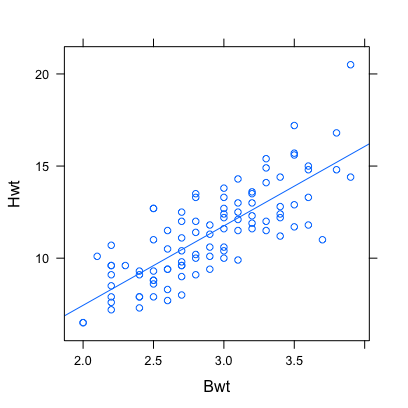Figure 1.1: Heart weight versus body weight of 97 male cats. A regression line has been fitted.

The fitted regression equation is:

mcats.lm <- lm(Hwt~Bwt, data=mcats)
signif(coef(summary(mcats.lm)),3)
            Estimate Std. Error t value Pr(>|t|)
(Intercept)    -1.18      0.998   -1.19 2.39e-01
Bwt             4.31      0.340   12.70 3.64e-22

Figure 1.2 shows the normal Q-Q plot of residuals. There are clear small and large outliers, though neither are widely removed from the main body of residuals. Also, the distribution is positively skew, as indicated by the bend in the Q-Q plot at a theoretical quantile of 0.

res <- resid(mcats.lm)
qqnorm(res)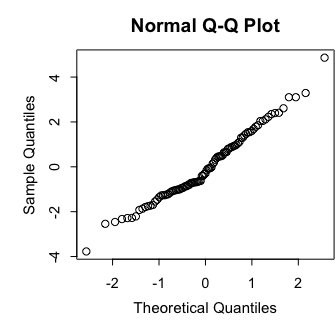Figure 1.2: Q-Q plot (‘Normal Probability plot’) of residuals from the regression of heart weight on body weight, for the male cats.

For later reference, determine the numbers of the rows that generated the ‘outliers’:

nout <- (1:nrow(mcats))[c(which.min(res),which.max(res))]

Bootstrap methods may be useful when the distribution of residuals is strongly skew. The distribution in Figure 1.2 is, notwithstanding the ‘outliers’, close enough to symmetric that, with 97 data points, the sampling distributions of the regression coefficients can fairly safely taken as normal. Examination of the bootstrap distributions will be used to check this claim.

Calculations use the functions simreg() and bootreg() respectively, from the {} package. Both sets of calculations start by fitting a regression, then calculating fitted values and residuals. Then the following calculation is repeated 1000 times:

• For simulation take a sample of 97 values from a normal distribution with the same standard deviation as the residuals. For bootstrap sampling take a bootstrap sample of the residuals.
• Add simulated residuals (bootstrap residuals) on to the fitted values, thus generating a new set of simulated (bootstrapped) $$y$$-values.
• Using the new simulated (bootstrapped) $$y$$-values fit a regression and record the coefficients.

Figure 1.3 shows the bootstrap results: Notice the strong correlation between Slope and Intercept. The marginal boxplots give a rough summary of the distributions:

library(gamclass, quietly=TRUE)
library(car, quietly=TRUE)
mcats <- subset(MASS::cats, Sex=="M")
bootmat <- bootreg(Hwt ~ Bwt,
data = mcats,
nboot = 1000)
bootdf <- as.data.frame(bootmat)
names(bootdf) <- c("Intercept",
"Slope")
colr <- adjustcolor(rep("black",3),
alpha.f=0.25)
scatterplot(Slope ~ Intercept,
col=colr, data=bootdf,
boxplots="xy",
regLine=NA,
smooth=FALSE)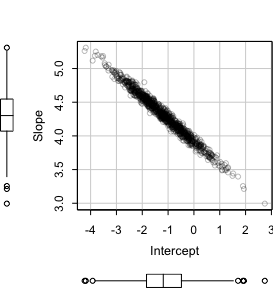Figure 1.3: Scatterplot matrix of bootstrap estimates of Slope versus Intercept, with boxplots used to summarize the marginal distributions.

Now calculate 95% confidence intervals for the intercept and slope:

sapply(bootdf, quantile, prob=c(.025, .975))
      Intercept Slope
2.5%    -3.0363 3.635
97.5%    0.8151 4.934

Bootstrap samples can include 0, 1, 2 or more repeats of row 97 that as identified as an outlier.

It is then instructive to compare Figure 1.3 with Figure 1.4, which shows the equivalent plots when the outlier is omitted:

• In Figure 1.4A the range of values, both on the Intercept and on the Slope scale, is reduced relative to Figure 1.3.
• Figure 1.4B shows the counterpart of Figure 1.3, from the use of simulation. The simulation process assumes a normal distribution, without outliers. The standard deviation used is however for the data that included the outlier. The range of values, on both axes, is accordingly greater than in 1.4A.
library(lattice)
bootmat <- bootreg(formula = Hwt ~ Bwt,
data = mcats[-97, ],
nboot = 1000)
bootdf0 <- as.data.frame(bootmat)
names(bootdf0) <- c("Intercept","Slope")
gphA <- xyplot(Slope ~ Intercept, data=bootdf0, alpha=0.25)
simmat <- simreg(formula = Hwt ~ Bwt,
data=mcats[-97, ], nsim=1000)
simdf <- as.data.frame(simmat)
names(simdf) <- c("Intercept","Slope")
gphB <- xyplot(Slope ~ Intercept, data=simdf, alpha=0.25)
update(gphA, main=grid::textGrob("A: Bootstrap (outlier omitted)",
x=grid::unit(.05, "npc"),
y = grid::unit(.25, "npc"), just="left",
gp=grid::gpar(cex=1)))
update(gphB, main=grid::textGrob("B: Simulation",
x = grid::unit(.05, "npc"),
y = grid::unit(.25, "npc"), just="left",
gp=grid::gpar(cex=1)))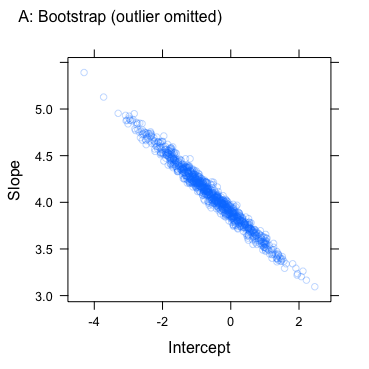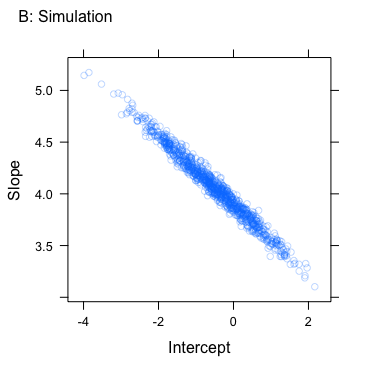Figure 1.4: Bootstrap resampling result, compared with simulation resampling.

# 2 Use tree-based methods appropriately!

cap5 <- "Regression tree for predicting Mileage given
Weight. At each node, observations for which the
criterion is satisfied take the branch to the left.  Thus,
at the first node, tonsWt$>=$1.218 chooses the branch to
the left, while tonsWt$<$1.218 chooses the branch to the
right.  Panel B plots Mileage versus tonsWt, with
fitted values from the rpart model shown as horizontal
grey lines."

The example now considered uses data, from the dataset rpart::cars, that are not well suited to the approaches implemented in the rpart and randomForest packages. Figure 2.1A, shows the regression tree from an rpart model that fits car Mileage as a function of tonsWt. The gray horizontal lines in Figure 2.1B show predictions from the model.

library(rpart)
Car90 <- na.omit(car90[, c("Mileage","Weight")])
## Express weight in metric tonnes
Car90 <- within(Car90, tonsWt <- Weight/2240)
car90.rpart <- rpart(Mileage ~ tonsWt, data=Car90)
## Code for Panel A
plot(car90.rpart)
text(car90.rpart, xpd=TRUE, digits=3)
mtext(side=3, line=1.25, "A: Regression tree", adj=0, cex=1.25)
## Code for Panel B
plot(Mileage ~ tonsWt, data=Car90, fg='gray')
wt <- with(Car90, tonsWt)
hat <- predict(car90.rpart)
gamclass::addhlines(wt, hat, lwd=2, col="gray")
mtext(side=3, line=1.25, "B: Predicted values from tree", adj=0, cex=1.25)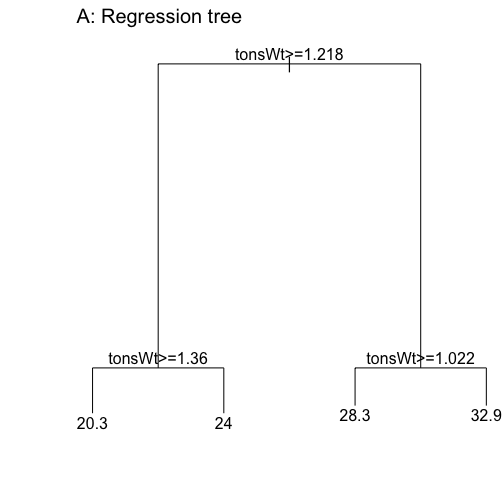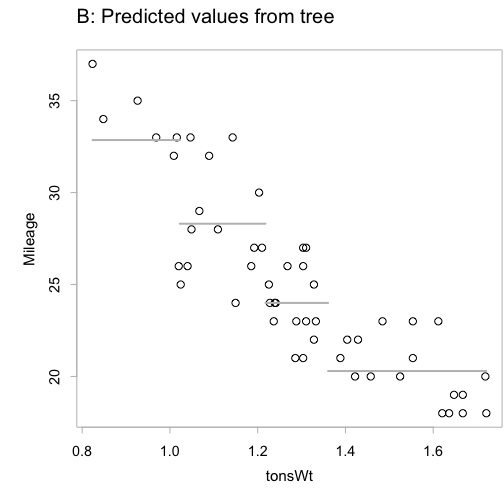Figure 2.1: Regression tree for predicting Mileage given Weight. At each node, observations for which the criterion is satisfied take the branch to the left. Thus, at the first node, tonsWt$>=$1.218 chooses the branch to the left, while tonsWt$<$1.218 chooses the branch to the right. Panel B plots Mileage versus tonsWt, with fitted values from the rpart model shown as horizontal grey lines.

The fitted values change in discrete units, where they should change smoothly. Least squares regression, fitting a line or curve is a much preferable tool for the task.

## 2.1 Choosing the split point

cap6 <- "Between group sum of squares for Mileage, as a
function of the value of tonsWt at which the split is
made. The choice $c = 1.218$ maximizes the between groups
sum of squares."

In the example used here, with just the one variable tonsWt, all splits are on a value of that variable. For each cutpoint $$c$$, with observations for values with $$x < c$$ placed in the first group, and observations for values of $$x >= c$$ placed in the second group, the split will partition the observations into two sets, with subscripts that we write $${j1}$$ and $${j2}$$. The between group sum of of squares for $$y$$ = Mileage is then: $BSS = n_1 (\bar{y_1} - \bar{y})^2 + n_2 (\bar{y_2} - \bar{y})^2$ where $$\bar{y_1}$$ is the mean for the $$n_1$$ values in the first group, $$\bar{y_2}$$ is the mean for the $$n_2$$ values in the second group, and $$\bar{y}$$ is the overall mean. The cutpoint $$c$$ that maximizes the $$BSS$$ is then chosen for the first split.

Figure 2.2 calculates and plots $$BSS$$ for all choices of cutpoint $$c$$, making it clear that the first split should be at tonsWt>=1.218.

## Code
BSS <- bssBYcut(tonsWt, Mileage, Car90)
with(BSS, plot(xOrd, bss, xlab="Cutpoint", fg='gray', ylab=""))
mtext(side=2, line=1.5, "Between groups\nsum of squares")
abline(v=1.218, lty=2)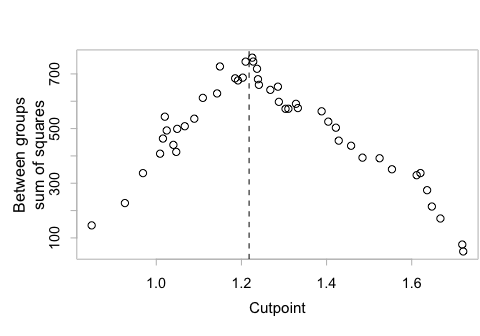Figure 2.2: Between group sum of squares for Mileage, as a function of the value of tonsWt at which the split is made. The choice $$c = 1.218$$ maximizes the between groups sum of squares.

# 3 Random forest fit to residuals from a trend surface

cap7 <- "Scatterplot matrix of accuracies for the several models.
The line $y=x$ is shown in each panel.
Note that rfOOB is out-of-bag accuracy, i.e., calculated from
the set of 95 observations, and that rfTest is accuracy on the
test data, again for a random forest model with no preliminary
smoothing. Results from hybrid models are labeled according to
the name of the formula for the smooth.   The final accuracy,
evaluated on the test data, is for a random forest model
fitted to residuals from the smooth"

A hybrid model may do better than, as investigated here, separate random forest or generalized additive models. The function gamclass::gamRF() fits a randomForest model for use as baseline, then also a hybrid model that follows use of the mgcv::gam() to fit a trend surface followed by use of randomForest() to fit to the residuals.

The dataset sp::meuse holds data on heavy metal concentrations at 155 locations near the village of Stein in the Netherlands, in the floodplain of the river Meuse. The best candidates for the initial trend surface will in general be variables that may plausibly have similar effects irrespective of geographical location, i.e., the variables dist, elev, ffreq and soil. The usefulness of including also x and y in the initial smooth can be checked. All variables from this set of five will then be used for the randomForest() fit to the residuals. The following model formulae for the initial smooth canvass a range of possibilities:

Repeated random subsets will be taken that comprise 95 of the 155 observations. For each such sample, the remaining 60 observations will be used for testing. These form just under 39% of the total, which is close to the just under the average 37% of the observations that each tree, from the fit to the total dataset, sets aside as test or out-of-bag’’ (OOB) data.

data("meuse", package="sp")
meuse <- within(meuse, {levels(soil) <- c("1","2","2")
ffreq <- as.numeric(ffreq)
loglead <- log(lead)
})
form1 <- ~ dist + elev + soil + ffreq
form3 <- ~ s(dist, k=3) + s(elev,k=3) + soil +ffreq
form3x <- ~ s(dist, k=3) + s(elev,k=3) + s(x, k=3) + soil+ffreq
form8x <- ~ s(dist, k=8) + s(elev,k=8) + s(x, k=8) + soil+ffreq
formlist <- list("Hybrid1"=form1, "Hybrid3"=form3, "Hybrid3x"=form3x,
"Hybrid8x"=form8x)
rfVars <- c("dist", "elev", "soil", "ffreq", "x", "y")
nrep <- 100
errsmat <- matrix(0, nrep, length(formlist)+2)
dimnames(errsmat)[] <- c(names(formlist), "rfTest", "rfOOB")
n <- 95
for(i in 1:nrep){
sub <- sample(1:nrow(meuse), n)
meuseOut <- meuse[-sub,]
meuseIn <- meuse[sub,]
errsmat[i, ] <- gamclass::gamRF(formlist=formlist, yvar="loglead",
rfVars=rfVars, data=meuseIn,
newdata=meuseOut, printit=FALSE)
}
## Code for scatterplot of results
ran <- range(errsmat)
at <- round(ran+c(0.02,-0.02)*diff(ran),2)
lis <- list(limits=ran, at=at, labels=format(at, digits=2))
lims=list(lis,lis,lis,lis,lis,lis)
library(lattice)
splom(errsmat,
pscales=lims,
par.settings=simpleTheme(cex=0.75),
col=adjustcolor("black", alpha=0.5),
panel=function(x,y,...){lpoints(x,y,...)
panel.abline(0,1,col="gray")}
)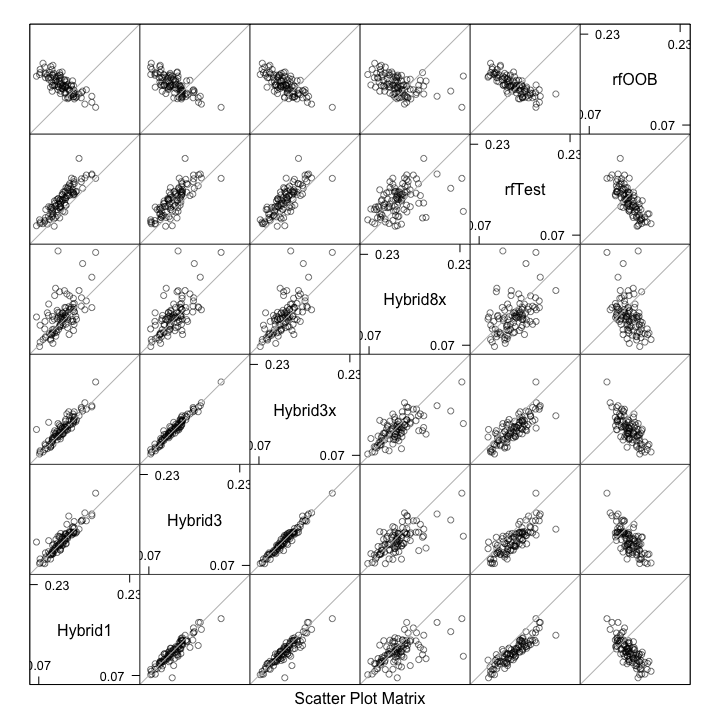Figure 3.1: Scatterplot matrix of accuracies for the several models. The line $$y=x$$ is shown in each panel. Note that rfOOB is out-of-bag accuracy, i.e., calculated from the set of 95 observations, and that rfTest is accuracy on the test data, again for a random forest model with no preliminary smoothing. Results from hybrid models are labeled according to the name of the formula for the smooth. The final accuracy, evaluated on the test data, is for a random forest model fitted to residuals from the smooth

errdf <- stack(as.data.frame(errsmat[,-6]))
names(errdf) <- c("errs","model")
errdf$model <- relevel(errdf$model, ref="rfTest")
errdf$sample <- factor(rep(1:nrow(errsmat),5)) errors.aov <- aov(errs ~ model+sample, data=errdf) round(coef(summary.lm(errors.aov))[1:5,], 4)  Estimate Std. Error t value Pr(>|t|) (Intercept) 0.1529 0.0059 26.024 0 modelHybrid1 -0.0189 0.0018 -10.373 0 modelHybrid3 -0.0165 0.0018 -9.076 0 modelHybrid3x -0.0149 0.0018 -8.156 0 modelHybrid8x -0.0096 0.0018 -5.276 0 model.tables(errors.aov, type="mean", cterms='model') Tables of means Grand mean 0.1188 model model rfTest Hybrid1 Hybrid3 Hybrid3x Hybrid8x 0.1308 0.1119 0.1142 0.1159 0.1211  # 4 Linear and quadratic discriminant analysis Comparisons are made with other methods also. cap8 <- "Length versus breadth, compared between cuckoo eggs laid in the different host nests. Axes for the scores are overlaid." cap9 <- "Plot of length versus breadth of cuckoo eggs, now showing a boundary line for distinguishing wren from not-wren, based on the lda() analysis." The dataset DAAG::cuckoos has measurements, from museum collections in the UK, of the length and breadth of eggs of each of six host species. What support does the data provide for the suggestion that, in nests of some host species at least, cuckoos match the dimensions of their eggs to those of the host species? Because there are just two measurements, a two-dimensional representation provides a complete description of the results of the analysis. Any plot of scores will be a rotated version of the plot of length versus breadth. The following uses to fit a model: cuckoos <- within(DAAG::cuckoos, levels(species) <- abbreviate(levels(species), 8)) cuckoos.lda <- MASS::lda(species ~ length + breadth, data=cuckoos) The list element cuckoos.lda$scaling gives the coefficients that are used in calculating the discriminant scores. The entries in the column headed LD1 are the coefficients of length and breadth that give the first set of discriminant scores. Those in the column headed LD2 give the second set of discriminant scores:

Code that can be used to give the scores is then: <<calc-scores, eval=TRUE, echo=TRUE>>= @ % The scores can be obtained directly from the calculation predict(cuckoos.lda)\$x. The following uses leave-one-out cross-validation to give accuracy assessments for lda(): gph <- xyplot(length ~ breadth, groups=species, data=cuckoos, type=c("p"), auto.key=list(space="right"), aspect=1, scales=list(tck=0.5), par.settings=simpleTheme(pch=16)) ## library(latticeExtra) # This package has the function layer() LDmat <- cuckoos.lda$scaling
ld1 <- LDmat[,1]
ld2 <- LDmat[,2]
gm <- sapply(cuckoos[, c("length", "breadth")], mean)
av1 <- gm + ld1/ld1*gm
av2 <- gm + ld2/ld2*gm
addlayer <- latticeExtra::layer(panel.abline(av1, -ld1/ld1, lty=1),
panel.abline(av2, -ld2/ld2, lty=2))
gph + addlayer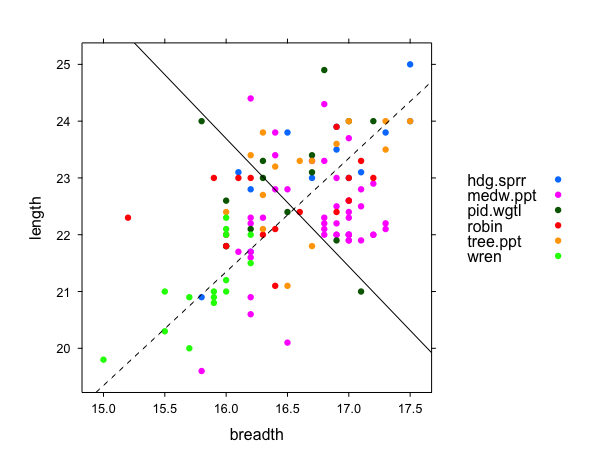Figure 4.1: Length versus breadth, compared between cuckoo eggs laid in the different host nests. Axes for the scores are overlaid.

gm <- apply(cuckoos.lda$means*cuckoos.lda$prior,2,sum)
# Grand 'means'
## Sweep out grand 'means'
centered <- sweep(as.matrix(cuckoos[,1:2]), 2, gm)
## Leave-one-out cross-validation
## Accuracies for linear discriminant analysis
cuckooCV.lda <- MASS::lda(species ~ length + breadth,
data=cuckoos, CV=TRUE)
confusion(cuckoos$species, cuckooCV.lda$class,
gpnames=abbreviate(levels(cuckoos$species), 8)) Overall accuracy = 0.433 Confusion matrix Predicted (cv) Actual hdg.sprr medw.ppt pid.wgtl robin tree.ppt wren hdg.sprr 0.000 0.571 0.143 0.071 0.143 0.071 medw.ppt 0.000 0.867 0.067 0.000 0.022 0.044 pid.wgtl 0.067 0.467 0.200 0.067 0.067 0.133 robin 0.000 0.625 0.188 0.000 0.062 0.125 tree.ppt 0.067 0.667 0.200 0.067 0.000 0.000 wren 0.000 0.267 0.000 0.067 0.000 0.667 ## Post-multiply by scaling matrix scores <- centered %*% cuckoos.lda$scaling

Here for comparison are accuracy assessments, again based on leave-one-out cross-validation, for qda(). The code is an obvious modification of that for lda():

## Accuracies for quadratic discriminant analysis
cuckooCV.qda <- MASS::qda(species ~ length + breadth,
data=cuckoos, CV=TRUE)
acctab <-confusion(cuckoos$species, cuckooCV.qda$class,
gpnames=levels(cuckoos$species), printit=FALSE)$confusion
tab <- table(cuckoos$species) ## ## Overall accuracy cat("Overall accuracy =", sum(diag(acctab)*tab)/sum(tab), "\n") Overall accuracy = 0.425  ## Confusion matrix round(acctab, 3)  Predicted (cv) Actual hdg.sprr medw.ppt pid.wgtl robin tree.ppt wren hdg.sprr 0.214 0.429 0.143 0.071 0.000 0.143 medw.ppt 0.000 0.822 0.044 0.000 0.044 0.089 pid.wgtl 0.067 0.533 0.067 0.067 0.133 0.133 robin 0.000 0.688 0.188 0.000 0.000 0.125 tree.ppt 0.200 0.600 0.133 0.067 0.000 0.000 wren 0.067 0.133 0.000 0.133 0.000 0.667 Notice that accuracy is slightly reduced, relative to the use of lda(). The calculations that follow will require an lda() model with CV=FALSE, which is the default: cuckoos.lda <- MASS::lda(species ~ length + breadth, data=cuckoos) x <- pretty(cuckoos$breadth, 20)
y <- pretty(cuckoos$length, 20) Xcon <- expand.grid(breadth=x, length=y) cucklda.pr <- predict(cuckoos.lda, Xcon)$posterior

Figure 4.2 shows contours for distinguishing wren from not-wren, for the lda() analysis (gray line).

m <- match("wren", colnames(cucklda.pr))
ldadiff <- apply(cucklda.pr, 1, function(x)x[m]-max(x[-m]))
addlayer1 <- latticeExtra::as.layer(contourplot(ldadiff ~ breadth*length,
at=c(-1,0,1), labels=c("", "lda",""),
label.style="flat", col='gray',
data=Xcon), axes=FALSE)
gph + addlayer1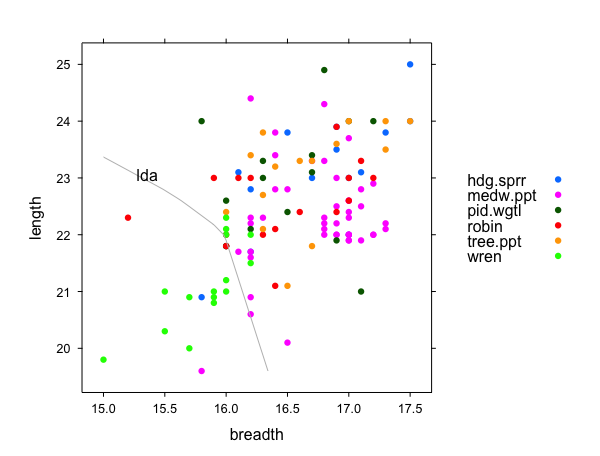Figure 4.2: Plot of length versus breadth of cuckoo eggs, now showing a boundary line for distinguishing wren from not-wren, based on the lda() analysis.

The function gamclass::compareModels() can be used to compare the accuracies of alternative model fits, checking for consistency over the data as a whole. Two model fits will be compared – the lda() fit above, and a variation on the lda() fit that includes terms in length^2, breadth^2 andlength*breadth.

library(gamclass, quietly=TRUE)
cucklda.pr <- cuckooCV.lda$posterior cucklda.pr2 <- MASS::lda(species ~ length + breadth + I(length^2) + I(breadth^2) + I(length*breadth), CV=TRUE, data=cuckoos)$posterior
compareModels(groups=cuckoos$species, estprobs=list(lda=cucklda.pr, "lda plus"=cucklda.pr2), gpnames=abbreviate(levels(cuckoos$species),8))
 "Average accuracies for groups:"
hdg.sprr medw.ppt pid.wgtl    robin tree.ppt     wren
0.1750   0.5113   0.1466   0.1525   0.1573   0.5513
Approx sed
0.0341
 "Average accuracies for methods:"
lda lda plus
0.3300   0.3488
Approx sed
0.0073 

The fit using qda(), which could also be included, has for simplicity been left out.

# 5 Tree-based methods

The dataset spam, from the kernlab package, was put together in 1999, with information on 2788 non-spam emails and 1813 spam emails. The emails are not dated. In the absence of more recent data from comparable sources that uses the same measures, there is no ready way to test how any model that might be developed would perform as one moves forward in time from 1999. Use of a holdout data set against which to assess results is the best that we can do.

A first step is to divide the dataset into 3 parts, thus:

data(spam, package='kernlab')
spam[,-58] <- scale(spam[,-58])
nr <- sample(1:nrow(spam))
spam0 <- spam[nr[1:2601],]      ## Training
spam1 <- spam[nr[2602:3601],]   ## Holdout
spam01 <- spam[nr[1:3601],]     ## Use for training,
## if holdout not needed
spam2 <- spam[nr[3602:4601],]   ## Test
library('MASS')
spam01.lda <- lda(type~., data=spam01)
ldaRates <- ldaErr(train.lda=spam01.lda, train=spam01, test=spam2,
group='type')

For calculation of the test error rate, the prior is set to the proportions in the training data spam01. This ensures that accuracies are for the same population mix of non-spam and spam.

Now use the function rpartErr() for a similar exercise for an rpart() model, and gamclass::rfErr() for a randomForest() model.

set.seed(29)   ## Make results precisely reproducible
spam01.rp <- rpart::rpart(type~., data=spam01, cp=0.0001)
rpRates <- rpartErr(train.rp=spam01.rp, train=spam01, test=spam2,
group='type')
set.seed(29)   ## Make results precisely reproducible
spam01.rf <- randomForest::randomForest(type ~ ., data=spam01)
rfRates <- rfErr(train.rf=spam01.rf, train=spam01, test=spam2,
group='type')

The following table summarizes results:

lda_main <- c(round(ldaRates['loo'], 3), round(ldaRates['trainerr'], 3),'-',
round(ldaRates['testerr'], 3))
rpartAcc <- c('-',round(rpRates['cverror'], 3),round(rpRates['trainerror'], 3),
round(rpRates['testerror'], 3))
rfAcc <- c('-',round(rfRates['trainerr'], 3),round(rfRates['OOBerr'], 3),
round(rfRates['testerr'], 3))
accmat <- rbind(lda_main,rpartAcc,rfAcc)
rownames(accmat) <- c('lda (main effects)','rpart','randomForest')
kable(accmat, col.names=c("Leave One Out CV","Training",
"Out of Bag","Test rate"), align=rep('r',4))
Leave One Out CV Training Out of Bag Test rate
lda (main effects) 0.116 0.112 - 0.119
rpart - 0.083 0.056 0.098
randomForest - 0.004 0.047 0.054

Calculation of a ten-fold cross-validation error rate for linear discriminant analysis is left as an exercise for the user. As the test data are a random sample from the total dataset, it should be similar to the test error rate.

## 5.1 Inclusion of interaction terms, with lda()

Can we improve model performance by including first order interaction terms? Factor by factor interaction terms allow a different estimate for each different combination of factor levels. Factor by variable interaction terms allow for a different slope for each different factor level. Variable by variable interaction terms create variables that are elementwise multiples of the two individual variables. The separate linear effects of variables x1 and x2 are increased or decreased by an amount that depends on the extent to which x1 and x2 increase or decrease together. In this example, with 57 explanatory variables, the effect is to generate a model matrix with 1653 columns. Main effects account for 57 columns, to which is added the choose(57,2) = 1596 ways of choosing combinations of two of the columns. The model matrix that results is (pretty much inevitably) multicollinear, over-fitting is likewise likely, higher order interactions are not accounted for, and calculations take a long time.

It is nevertheless insightful to proceed with the (time-consuming) fit, and note the results:

mm01x <- model.matrix(type ~ 0+.^2, data=spam01)
mm2x <- model.matrix(type ~ 0+.^2, data=spam2)
spam01x.lda <- lda(x=mm01x, grouping=spam01[,'type'])
ldaRatesx <- ldaErr(train.lda=spam01x.lda, train=mm01x, test=mm2x,
group=spam01[,'type'])
round(ldaRatesx,3)

Results obtained were:

     loo trainerr  testerr
0.291    0.027    0.164 

The test error rate is the standard against which the other two rates should be compared. The leave-one-out estimate is wildly astray. A training rate that is highly over-optimistic is not surprising, given the large number of explanatory terms.

The big advantage of randomForest() is its ability to accommodate what, using classical approaches, would require the incorporation of high order interaction terms.

## 5.2 Multiple Levels of variation – the Vowel dataset

The discussion that follows is designed to illustrate the important point that estimates of prediction error must, to be useful, reflect the intended generalization. For inference from the data considered here, prediction for one of the 15 speakers represented in the data is an inherently easier task than prediction for a new speaker.

The dataset Vowel (in the mlbench package) has data on 15 different speakers, each tested 6 times on each of 11 different vowels.
Information additional to the dataset’s help page can be found at http://archive.ics.uci.edu/ml/index.php.

data('Vowel', package='mlbench')
Vowel$V1 <- factor(Vowel$V1)

The first data column identifies the speaker/vowel combination. The next 9 columns of the data are measures derived from auditory signal processing. The final column identifies the vowel sound. The V1 identifies the speaker. For prediction for a new speaker, V1 is not a useful explanatory factor.

We will compare the following:

• lda(), without and with first order interactions
• qda()
• randomForest()

Except for randomForest(), we will use cross-validation with subjects as clusters to assess accuracy, i.e., subjects will be left out one at a time.^[Very similar results are obtained if they are left out three at a time, i.e., 5 folds]. For randomForest(), 500 bootstrap samples of subjects will be taken. For each bootstrap sample, a single tree will be found, and predictions made for the out-of-bag subjects. This is a clumsy use of randomForest(), necessary however because randomForest() does not have provision for sampling the data in a manner that reflects the intended generalization. For prediction to new speakers, each tree must be based on a bootstrap sample of speakers, with estimated error rates based on performance for OOB speakers.

vowelcv.qda <- qda(Class ~ ., data=Vowel, CV=TRUE)
accqda <- confusion(Vowel$Class, vowelcv.qda$class,
printit=FALSE)$overall print(round(accqda,3))  0.984 ## Compare with CV performance accspqda <- CVcluster(Class ~ ., id=V1, data=Vowel, nfold=15, FUN=qda, printit=FALSE)$CVaccuracy
print(round(accspqda,3))
 0.467
data('Vowel', package='mlbench')
Vowel$V1 <- factor(Vowel$V1)
vowel.rf <- randomForest::randomForest(Class ~ ., data=Vowel)
print("OOB accuracy estimate")
 "OOB accuracy estimate"
accOOB <- 1-sum(rep(1/11,11)*vowel.rf$confusion[,"class.error"]) print(round(accOOB,3))  0.98 ## Compare with performance based on OOB choice of speakers accspOOB <- RFcluster(Class ~ ., id=V1, data=Vowel, ntree=500, progress = FALSE)$OOBaccuracy
OOB accuracy = 0.56 \n
print(round(accspOOB),3)
 1